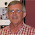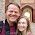## Sunday, November 25, 2012

### Commodity Demand Estimation - Fish Demand

Stata Do File

* My field exam is coming up and I am therefore giving some thought to commodity demand once again.

* This simulation will follow the Paper "Demand Structure for Fish" Asche, Bjorndal, and Gordan SNF Working Paper No. 37/05

* In this simulation I will attempt to simulate commodity data that fits several empirical model specifications.

* A common initial attempt at estimating demand was to specify:

* ln(q_it) = alpha_i + sum(across j) of Beta_j*ln(pjt) + e_i*ln(X_t)

* The advantage of this formation was that it allowed the elasticities to be easily recovered.

* If consumers of fish have a fixed budget for fish and no strong substitutes then we expect that the price elasticity of fish to be around -1.

* Let's see if we can generate aggrogate data that looks like this.

clear
* Let's say we have 20 years of weekly price data ~1000 observations
set obs 1000
gen t=_n

* Let's generate some substitute products
gen pbeef = (rpoisson(10)+1)/10
gen pchicken = (rpoisson(7)+1)/10
label var pchicken "Price of Chicken"
gen ppork = (rpoisson(12)+1)/14
gen pfish = (rpoisson(15)+1)/12
label var pfish "Price of Fish"

* Let's generate some fish covariates.

* There was a scare that commercial fishing was killing dolphins.  Let's say that happened at time 500.
gen dolphin = 0
replace dolphin = 1 if t>500

* Let's imagine that the fishing industry is also campainging to encourage the consumption of fish.
gen fishad = rnbinomial(10,.1)

* Now let's generate the natural logs of the above values
foreach v in pbeef pchicken ppork pfish fishad {
gen lnv' = ln(v')
}

* Generate an error term
gen e=rnormal()/4

* Now let's generate quantity demand for fish!
gen lnqfish = 1 + .3*lnpbeef + .5*lnpchicken + .3*lnppork - 1*lnpfish - .3*dolphin + .1*lnfishad + e

* Done with simulation

* I won't even bother estimating this. The reason is that by construction our data perfectly fits our OLS model.

* Well, just a reference point I will estimate the above.
reg lnqfish lnpbeef lnpchicken lnppork lnpfish dolphin lnfishad

* We can see all of our estimates work exceedingly well when the model is perfectly specified.

* However, it might be instructive to look at the relationships between variables.

gen qfish = exp(lnqfish)
label var qfish "Quantity of Fish Purchased"

twoway (scatter pchicken qfish) (lfit pchicken qfish) ///
(scatter pfish qfish) (lfit pfish qfish) ,     ///
title(Relationship Between Own Price and Quantity)

* This graph is a little bit busy and might be hard to read.
* Notice that each point in blue is also represented in green.
* This is because while the horizontal axis is the same the vertical axis changes from price of chicken to price of fish.

* Let's imagine that rather than estimating using logs we estimated using quantities and prices.
reg qfish pbeef pchicken ppork pfish dolphin fishad

* It is interesting to note that while the estimates when the functional form is not speficied correctly are not as good as previously they still seem to be working well.

* Let's try converting some of them to point elasticities and see how they compare.

foreach v in pbeef pchicken ppork pfish fishad {
qui sum v'
di "Point Elasticity of v' = =_b[v']/r(mean)'"
}

* Some of the point elasticities are better estimates of the constant elasticity than just the coefficients but not consistently so.

* Okay, so this has gotten our feet a little wet with the idea of estimating demand.
* Now, let's shift into demand estimation given a more flexible forms for the demand to take.

*****************************************************
* Houthakker and Taylor's habit formation model.

* ln(q_it) = alpha_i + c_i*ln(q_i,t-1) + sum(across j) of Beta_j*ln(pjt) + e_i*ln(X_t)

* The difference between this model and the last is that now we have a form of temporal dependence.

* Consumption this period depends upon consumption last period.

gen lnqfish2 = 1 + .75*lnpbeef + .5*lnpchicken + .3*lnppork - 1*lnpfish - .3*dolphin + .1*lnfishad + e if t==1
replace lnqfish2 = .75*lnqfish2[_n-1] + .3*lnpbeef + .5*lnpchicken + .3*lnppork - 1*lnpfish - .3*dolphin + .1*lnfishad + e if t>1
label var lnqfish2 "Auto-correlated Fish Quantity Demanded"

* In order to see the time trends
forv i=2/12 {
gen ti'=t^(i'/3)
}

reg lnqfish2 t t? t??

predict lnqfish2_hat

two (line lnqfish2 t) (line lnqfish2_hat t) , title(Fish Quantity Demanded with Temporal Dependency)

* We can see long term movement in quantity demanded as a result of the dophin scare in additition to some short term temporal dependency as a result of habit formation.
gen lnqfish2_l = lnqfish2[_n-1]

* To verify that we can estimate the model directly:
reg lnqfish2 lnqfish2_l lnpbeef lnpchicken lnppork lnpfish dolphin lnfishad

* As before, when our model is correctly specified we have little difficulty estimating the parameters.

* If we fail to include the once lagged fish quantity:
reg lnqfish2 lnpbeef lnpchicken lnppork lnpfish dolphin lnfishad
* We have less explanatory power, but generally almost all of the estimates are identical.

predict resid, resid

twoway (scatter resid lnqfish2) (lfit  resid lnqfish2)
* There is a clear relationship between the y variable and the residual suggesting autocorrelation.

* Even though there is autocorrelation of the errors this does not substantially affect the estimates of the demand function.

* However, this is somewhat an artifact of the simulation.

* We should think that there might be a causal relationship between amount of fish demanded during the previous period and current period prices if there exists some friction in the fish markets.

* Thus perhaps the higher the quantity demanded in the current period the higher the price is expected to be during the next period because of fish reserves.

* This demands a more complex simulation in which we calculate each period individually.

gen lnpfish2 = ln((rpoisson(15)+1)/12) if t==1
gen lnqfish3 = 1 + .3*lnpbeef + .5*lnpchicken + .3*lnppork - 1*lnpfish2 - .3*dolphin + .1*lnfishad + e if t==1

* For the first period we assume prices are exogenous.

* For each additional period we will make prices a function of quantity demanded.
forv i=2/1000 {
qui replace lnpfish2 = ln((rpoisson(15)+1)/12) + .7*lnqfish3[i'-1] if t==i'
* Thus the price elasticity is something less than 1/.7= 1.4.  Less because there is the random poisson variation that diminishes the relationship.
qui replace  lnqfish3 = .75*lnqfish3[_n-1] + .3*lnpbeef + .5*lnpchicken + .3*lnppork - 1*lnpfish2 - .3*dolphin + .1*lnfishad + e if t==`i'
}

reg lnqfish3 lnpbeef lnpchicken lnppork lnpfish2 dolphin lnfishad
* Now failing to take into account the previous quantity of fish demanded causes the price elasticity to be downward biased.

* The reason is that high quantity demanded last period will cause the habit of high quantity demanded this period.

* However, high quantity demanded last period will also cause the price to be higher this period.

* If you do not control for high demand last period then the higher price will look like it is explaining both the higher habitual consumption of fish and the lower consumption of fish due to the higher price.

* Thus, controlling for habit formation is necessary for unbiased estimates.
gen lnqfish3_l = lnqfish3[_n-1]
reg lnqfish3 lnqfish3_l lnpbeef lnpchicken lnppork lnpfish2 dolphin lnfishad

predict res3, residual

scatter res3 t if t<200 autocorrelation="autocorrelation" o="o" of="of" p="p" remaining="remaining" residuals="residuals" title="title">

gen res3_1 = res3[_n-1]

reg res3_1 res3
* After controlling for AR1 errors, there is no longer autocorrelation of residuals.

1.Francis - a (system of) demand function(s) needs to be consistent with maximizing an underlying utility fucntiopn. Unfortunately, there is no sensible utility function which, when maximized, yields a log-log demand function.

DG

1.Good point. This raises the essential question, "How wed are we to economic theory?" If we are simply interested in a forecasting model then perhaps we don't really care that our model is disconnected from theory so long as we can predict reasonably well.

However, there is a deeper question here as well. Do we restrict our predictive power because we do not have a theoretical model that fits the empirical model? It is easy to come up with examples for why empirical work is meaningless without a theoretical model. However, what happens when there is no reasonable available theoretical model and we are asked to answer a specific question?

I don't don't have an answer for any of this. I am sure a theoretical economists would have an easy answer. But, it might not be the best or the right one though the one which is logically consistent. For instance, the demand function that is consistent with utility theory. But, what happens if utility theory is an untrue yet useful concept.

Should we restrict our models to only those that fit within utility theory?

2.This comment has been removed by the author.